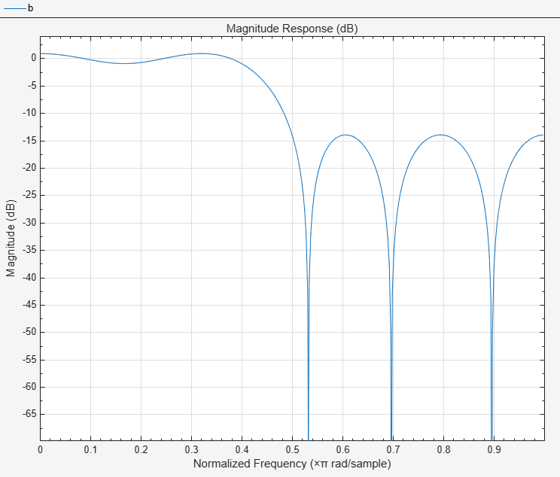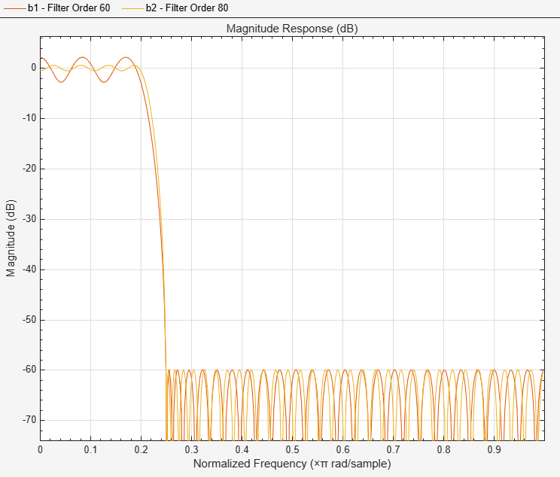fircband

Constrained-band equiripple FIR filter

Syntax

b = fircband(n,f,a,w,c)
b = fircband(n,f,a,s)
b = fircband(...,'1')
b = fircband(...,'minphase')
b = fircband(..., 'check')
b = fircband(...,{lgrid})
[b,err] = fircband(...)
[b,err,res] = fircband(...)

Description

fircband is a minimax filter design algorithm that you use to design the following types of real FIR filters:

• Types 1-4 linear phase

• Type 1 is even order, symmetric

• Type 2 is odd order, symmetric

• Type 3 is even order, antisymmetric

• Type 4 is odd order, antisymmetric

• Minimum phase

• Maximum phase

• Minimum order (even or odd), extra ripple

• Maximal ripple

• Constrained ripple

• Single-point band (notching and peaking)

• Forced gain

• Arbitrary shape frequency response curve filters

b = fircband(n,f,a,w,c) designs filters having constrained error magnitudes (ripples). c is a cell array of character vectors of the same length as w. The entries of c must be either 'c' to indicate that the corresponding element in w is a constraint (the ripple for that band cannot exceed that value) or 'w' indicating that the corresponding entry in w is a weight. There must be at least one unconstrained band — c must contain at least one w entry. For instance, the example 'Design a Constrained Lowpass Filter' uses a weight of one in the passband, and constrains the stopband ripple not to exceed 0.2 (about 14 dB).

A hint about using constrained values: if your constrained filter does not touch the constraints, increase the error weighting you apply to the unconstrained bands.

Notice that, when you work with constrained stopbands, you enter the stopband constraint according to the standard conversion formula for power — the resulting filter attenuation or constraint equals 20*log(constraint) where constraint is the value you enter in the function. For example, to set 20 dB of attenuation, use a value for the constraint equal to 0.1. This applies to constrained stopbands only.

b = fircband(n,f,a,s) is used to design filters with special properties at certain frequency points. s is a cell array of character vectors and must be the same length as f and a. Entries of s must be one of:

• 'n' — normal frequency point.

• 's' — single-point band. The frequency band is given by a single point. You must specify the corresponding gain at this frequency point in a.

• 'f' — forced frequency point. Forces the gain at the specified frequency band to be the value specified.

• 'i' — indeterminate frequency point. Use this argument when bands abut one another (no transition region).

b = fircband(...,'1') designs a type 1 filter (even-order symmetric). You could also specify type 2 (odd-order symmetric), type 3 (even-order antisymmetric), or type 4 (odd-order antisymmetric) filters. Note there are restrictions on a at f = 0 or f = 1 for types 2, 3, and 4.

b = fircband(...,'minphase') designs a minimum-phase FIR filter. There is also 'maxphase'.

b = fircband(..., 'check') produces a warning when there are potential transition-region anomalies in the filter response.

b = fircband(...,{lgrid}), where {lgrid} is a scalar cell array containing an integer, controls the density of the frequency grid.

[b,err] = fircband(...) returns the unweighted approximation error magnitudes. err has one element for each independent approximation error.

[b,err,res] = fircband(...) returns a structure res of optional results computed by fircband, and contains the following fields:

Structure Field

Contents

res.fgrid

Vector containing the frequency grid used in the filter design optimization

res.des

Desired response on fgrid

res.wt

Weights on fgrid

res.h

Actual frequency response on the frequency grid

res.error

Error at each point (desired response - actual response) on the frequency grid

res.iextr

Vector of indices into fgrid of external frequencies

res.fextr

Vector of extremely frequencies

res.order

Filter order

res.edgecheck

Transition-region anomaly check. One element per band edge. Element values have the following meanings: 1 = OK , 0 = probable transition-region anomaly , -1 = edge not checked. Computed when you specify the 'check' input option in the function syntax.

res.iterations

Number of Remez iterations for the optimization

res.evals

Number of function evaluations for the optimization

Examples

collapse all

Design a 12th-order lowpass filter with a constraint on the filter response.

b = fircband(12,[0 0.4 0.5 1], [1 1 0 0], ...
[1 0.2], {'w' 'c'});

Using fvtool to display the result b shows you the response of the filter you designed.

fvtool(b)Design two filters of different order with the stopband constrained to 60 dB. Use excess order (80) in the second filter to improve the passband ripple.

b1 = fircband(60,[0 .2 .25 1],[1 1 0 0],...
[1 .001],{'w','c'});
b2 = fircband(80,[0 .2 .25 1],[1 1 0 0],...
[1 .001],{'w','c'});
hfvt = fvtool(b1,1,b2,1);
legend(hfvt,'Filter Order 60','Filter Order 80');